# 1700 Time for a Horse Race!

### Today’s Puzzle:

I’ve made a table of all the numbers from 1601 to 1700, their prime factorizations, and how many factors each of those numbers has.Each number from 1601 to 1700 has 2, 3, 4, 6, 8, 10, 12, 16, 18, 24, 30 or 40 factors. Do more numbers have 2 factors, 3 factors, or a different number? Sure, you could use the table to count, but it will be more fun if we make a horse race out of it. What pony will you pick, 2, 3, 4, 6? Make your prediction of the winner, and then watch the horse race to see if you were right.

Here are all the horses lined up at the gate. Pick your pony!And they’re off!make science GIFs like this at MakeaGif
The first half of the race was VERY interesting to me. Three different horses held the lead, and the leaders in the race was even neck and neck some of the time. After you know the winner, watch the race again, following the winner from start to finish.

### Factors of the Number 1700:

• 1700 is a composite number.
• Prime factorization: 1700 = 2 × 2 × 5 × 5 × 17, which can be written 1700 = 2² × 5² × 17.
• 1700 has at least one exponent greater than 1 in its prime factorization so √1700 can be simplified. Taking the factor pair from the factor pair table below with the largest square number factor, we get √1700 = (√100)(√17) = 10√17.
• The exponents in the prime factorization are 2, 2, and 1. Adding one to each exponent and multiplying we get (2 + 1)(2 + 1)(1 + 1) = 3 × 3 × 2 = 18. Therefore 1700 has exactly 18 factors.
• The factors of 1700 are outlined with their factor pair partners in the graphic below.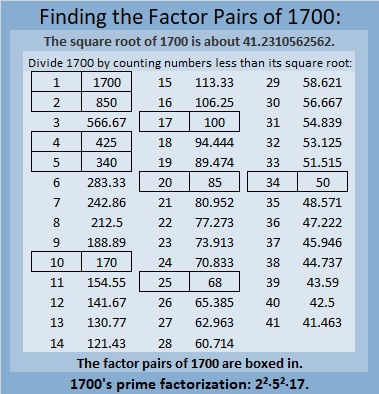### More About the Number 1700:

1700 is the sum of two squares THREE different ways:
40² + 10² = 1700,
38² + 16² = 1700, and
32² + 26² = 1700.

Those sum of two squares mean 1700 is the hypotenuse of some Pythagorean triples, SEVEN to be exact:

1. 260-1680-1700, which is 20 times (13-84-85),
2. 348-1664-1700, which is 4 times (87-416-425), but it can also be calculated from 32² – 26², 2(32)(26), 32² + 26²
3. 476-1632-1700, which is (7-24-25) times 68,
4. 720-1540-1700, which is 20 times (36-77-85),
5. 800-1500-1700, which is (8-15-17) times 100, but it can also be calculated from 2(40)(10), 40² – 10², 40² + 10²,
6. 1020-1360-1700, which is (3-4-5) times 340,
7. 1188-1216-1700, which is 4 times (297-304-425), but it can also be calculated from 38² – 16², 2(38)(16), 38² + 16².

As OEIS.org informs us, 1700 is a Catalan number. It is found in the C(13,4) position, as shown below. With the exception of the lone 1 in the 0th row of the triangle, every number in Catalan’s triangle is the sum of the number above it and the number to its left. For example, 1700 = 1260 + 440.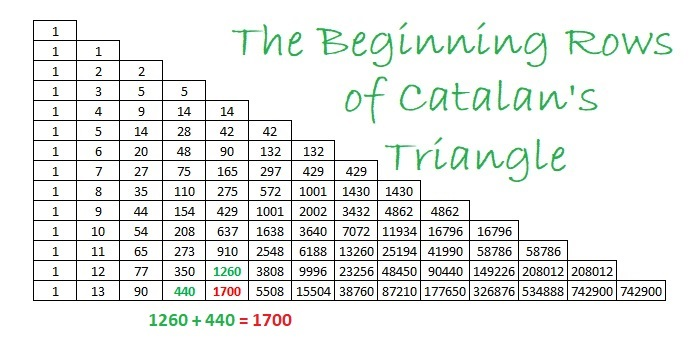I hope you have enjoyed learning about the number 1700.

# 1600 How Would You Describe This Horse Race?

### Today’s Puzzle:

Do most of the numbers from 1501 to 1600 have 2 factors, 4 factors, 6 factors, or what? A horse race is a fun way to find the answer to that puzzle!

As I’ve done several times before, I’ve made a horse race for this multiple of 100 and the 99 numbers before it. A horse moves when a number comes up with a particular amount of factors. Some of the races I’ve done in the past have been exciting with several lead changes. In other races, one horse ran quite quickly, leaving all other horses in the dust. One previous horse race resulted in a tie.

How will you describe this horse race? Exciting or boring? Surprizing or predictable? Pick your pony and watch the race to the end before you decide on an adjective.

Click here if you would like the Horse Race to be slightly bigger.make science GIFs like this at MakeaGif
Wow! I’ve not seen that happen before! Visually it looks like 4 won the race, but this horse race is really about finding the mode There are two modes, 4 and 8, for the amount of factors for the numbers from 1501 to 1600. It’s about which amount of factors comes up most often for the entire set of numbers, not which one of those occurs first. Thus, for that reason, I would describe the horse race above as deceptive. That horse race looked at the amount of factors five numbers at a time. Here’s what happens if we look at ten numbers at a time:
In the second horse race, it is much more clear that the race ends in a tie, and the mode is BOTH 4 and 8.

### Prime Factorization of Numbers from 1501 to 1600:Of those 100 numbers, 38 have square roots that can be simplified; 62 do not.

### Factor Trees for 1600:

1600 has MANY possible factor trees. Some are symmetrical; some are not. Here are two nicely shaped ones:### Factors of 1600:

• 1600 is a composite number and a perfect square.
• Prime factorization: 1600 = 2 × 2 × 2 × 2 × 2 × 2 × 5 × 5, which can be written 1600 = 2⁶ × 5².
• 1600 has at least one exponent greater than 1 in its prime factorization so √1600 can be simplified. Taking the factor pair from the factor pair table below with the same number for both factors, we get
√1600 = (√40)(√40) = 40. However, you could also use
√1600 = (√4)(√400) = 2 × 20 = 40,
√1600 = (√16)(√100) = 4 × 10 = 40, or
√1600 = (√25)(√64) = 5 × 8 = 40.
• The exponents in the prime factorization are 6 and 2. Adding one to each exponent and multiplying we get (6 + 1)(2 + 1) = 7 × 3 = 21. Therefore 1600 has exactly 21 factors.
• The factors of 1600 are outlined with their factor pair partners in the graphic below.### More about the Number 1600:

1600 is the sum of two squares:
32² + 24² = 1600.

1600 is the hypotenuse of two Pythagorean triples:
448-1536-1600, calculated from 32² – 24², 2(32)(24), 32² + 24².
It is also (7-24-25) times 64.
960-1280-1600, which is (3-4-5) times 320.

1600 looks square in some other bases:
1600 = 1(40²) + 0(40) + 0(1), so it’s 100₄₀.
1600 =1(39²) + 2(39) + 1(1), so it’s 121₃₉.
1600 =1(38²) + 4(38) + 4(1), so it’s 144₃₈.
1600 =1(37²) + 6(37) + 9(1), so it’s 169₃₇.

Furthermore, 1600 is a repdigit in base 7:
1600 = 4(7³ + 7² + 7¹ + 7º), so it’s 4444₇.

# Celebrating 1500 with a Horse Race and Much More!

Writing 1500 posts is quite a milestone. I’ll begin the celebration with an exciting horse race! Let me explain:

Each prime number has exactly 2 factors. Every composite number between 1401 and 1500 has somewhere between 4 and 36 factors. Which quantity of factors do you think will appear most often for these numbers? Pick that amount as your pony and see how far it gets in this horse race!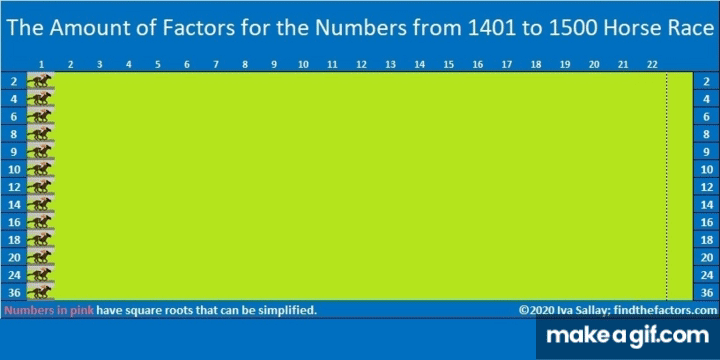make science GIFs like this at MakeaGif

Did the race results surprise you? They surprised me!

### Prime Factorization for numbers from 1401 to 1500:

Here’s a chart showing the prime factorization of all those numbers and the amount of factors each number has. Numbers in pink have exponents in their prime factorization so their square roots can be simplified:### Today’s Puzzles:

Let’s continue the celebration with a puzzle: 1500 has 12 different factor pairs. One of those pairs adds up to 85 and one of them subtracts to give 85. Can you find those factor pairs that make sum-difference and write them in the puzzle? You can look at all of the factor pairs of 1500 in the graphic after the puzzle, but the second puzzle is really just the first puzzle in disguise. So try solving that easier puzzle first.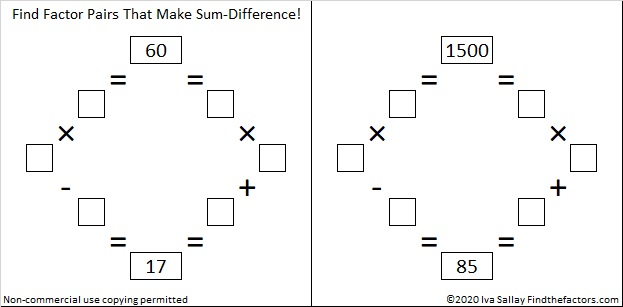### Factors of 1500:

• 1500 is a composite number.
• Prime factorization: 1500 = 2 × 2 × 3 × 5 × 5 × 5, which can be written 1500 = 2² × 3 × 5³
• 1500 has at least one exponent greater than 1 in its prime factorization so √1500 can be simplified. Taking the factor pair from the factor pair table below with the largest square number factor, we get √1500 = (√100)(√15) = 10√15
• The exponents in the prime factorization are 2, 1, and 3. Adding one to each exponent and multiplying we get (2 + 1)(1 + 1)(3 + 1) = 3 × 2 × 4 = 24. Therefore 1500 has exactly 24 factors.
• The factors of 1500 are outlined with their factor pair partners in the graphic below.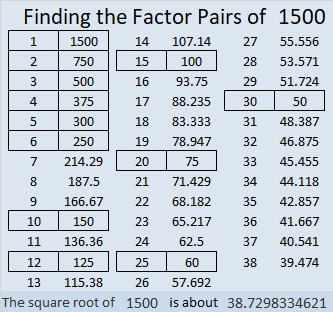### A Forest of Factor Trees:

Take a few minutes to hike in this forest featuring a few of the MANY possible factor trees for 1500. Celebrate each tree’s uniqueness!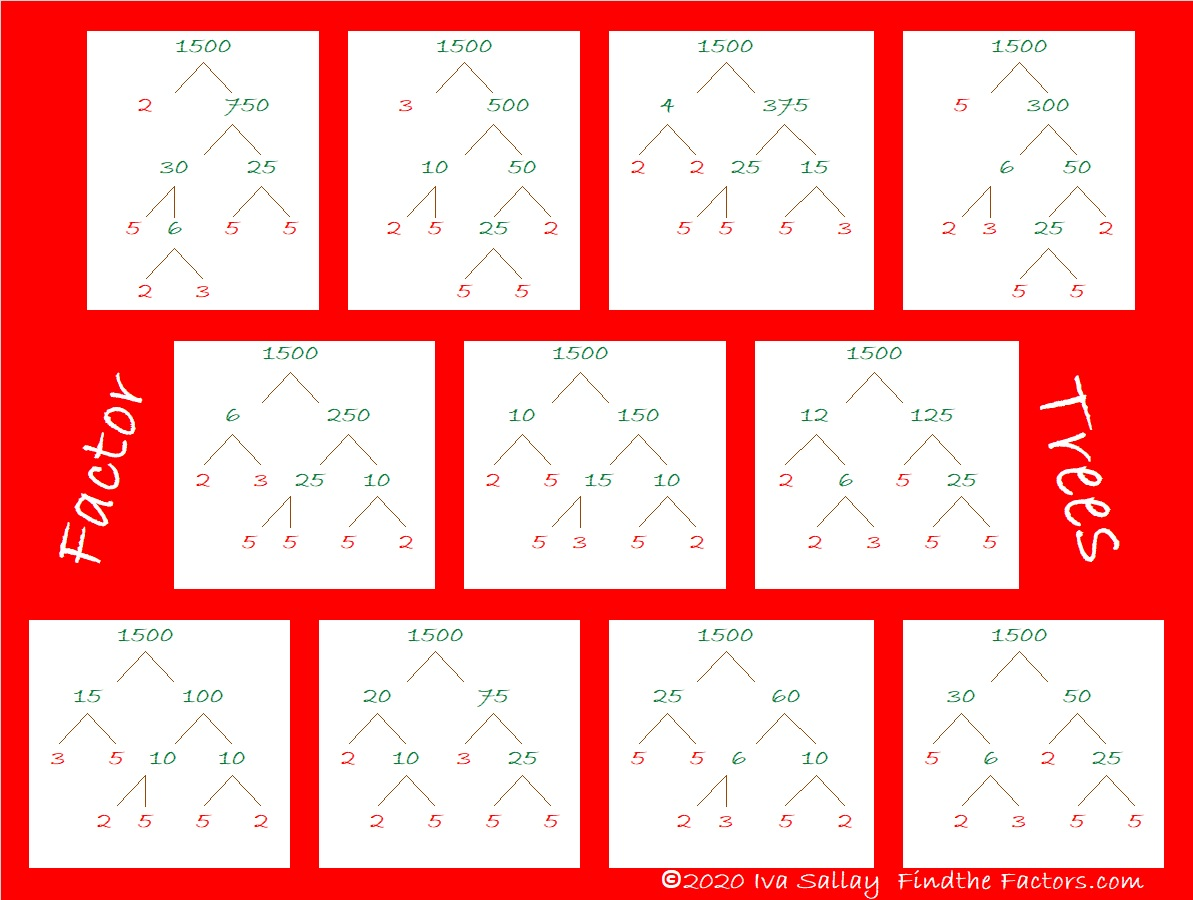### Other Facts to Celebrate about 1500:

Oeis.org tells us that  (5+1) × (5+5) × (5+0) × (5+0) = 1500.

1500 is the hypotenuse of THREE Pythagorean triples:
420-1440-1500, which is (7-24-25) times 60,
528-1404-1500, which is 12 times (44-117-225),
900-1200-1500, which is (3-4-5) times 300.

# 1100 Now THIS Is a Horse Race!

Most people know that any prime number has exactly 2 factors. Every composite number has more than 2 factors. I’ve made a chart showing the numbers from 1001 to 1100, their prime factorizations, and the amount of factors each of those numbers has.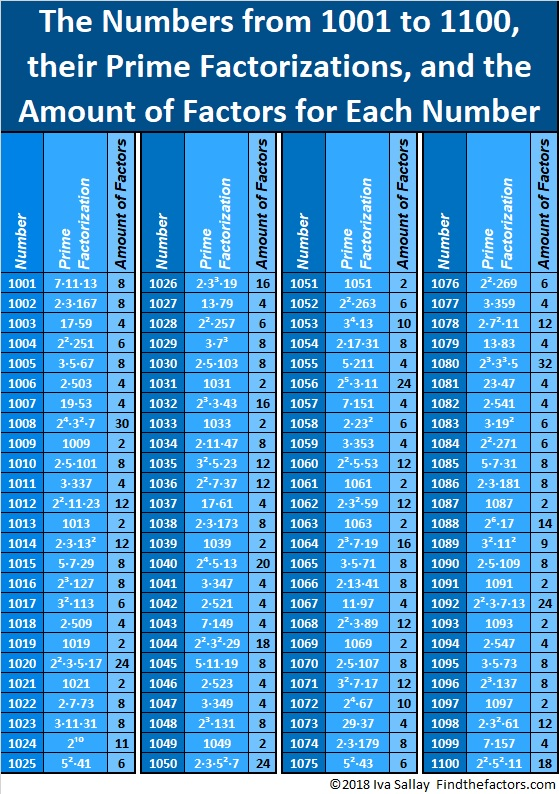The fewest factors any of those numbers have is 2 while the most factors any of the numbers have is 32. Which number of factors appears most often? Let’s have a horse race to find out!

Several horses representing different amounts of factors are lined up for the race. Spoiler alert: some of the horses will barely make it out of the gate. THIS will be an exciting horse race. There will be at least one lead change. You won’t know for sure which horse will cross the finish line first until the end. The second place horse will be SO close to winning.

I haven’t made a horse race this exciting since the 601 to 700 Horse Race, so pick your pony, then scroll down and see how your pony does!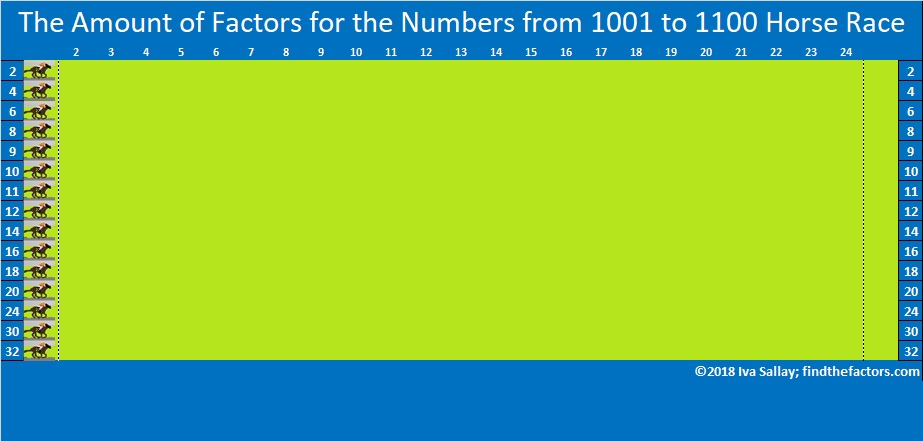Click on the gif below to make the horse race larger.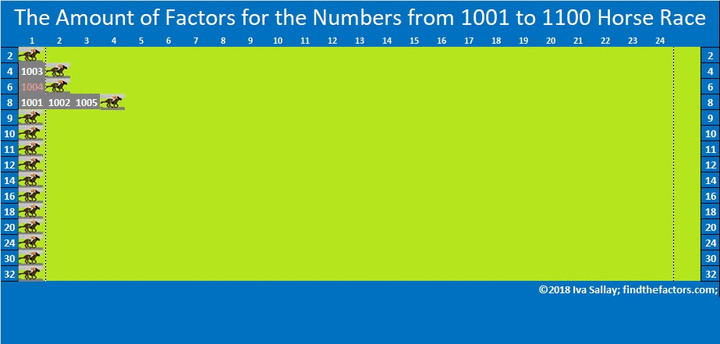make science GIFs like this at MakeaGif

How did your pony do? Were you surprised by the results of the race?

Only perfect squares can have an odd number of factors which explains why 9 and 11 barely make it out of the gate. The numbers in pink have square factors so their square roots can be simplified. That describes 41 of the numbers from 1001 to 1100.

I hope this horse race sparks your curiosity about numbers! Each number is fascinating in its own way.

Let me tell you a few things specifically about the number 1100:

• 1100 is a composite number.
• Prime factorization: 1100 = 2 × 2 × 5 × 5 × 11, which can be written 1100 = 2² × 5² × 11
• The exponents in the prime factorization are 2, 2 and 1. Adding one to each and multiplying we get (2 + 1)(2 + 1)(1 + 1) = 3 × 3 × 2 = 18. Therefore 1100 has exactly 18 factors.
• Factors of 1100: 1, 2, 4, 5, 10, 11, 20, 22, 25, 44, 50, 55, 100, 110, 220, 275, 550, 1100
• Factor pairs: 1100 = 1 × 1100, 2 × 550, 4 × 275, 5 × 220, 10 × 110, 11 × 100, 20 × 55, 22 × 50 or 25 × 44
• Taking the factor pair with the largest square number factor, we get √1100 = (√100)(√11) = 10√11 ≈ 33.166251100 is the hypotenuse of two Pythagorean triples:
660-880-1100 which is (3-4-5) times 220
308-1056-1100 which is (7-24-25) times 44

It may interest you to know that 1100 is 3131 in BASE 7.
That’s because 3(7³) + 1(7²) + 3(7) + 1( 1) = 1100.

All those fun facts are straight from the horse’s mouth!

# 900 Pick Your Pony. Who’ll Win This Amount of Factors Horse Race?

I really like this rhyme that I saw for the first time this week (even though it’s all over the net):

Hey diddle diddle, the median’s the middle,
You add then divide for the mean.
The mode is the one that appears the most,
And the range is the difference between.

All of the numbers from 801 to 900 have at least 2 factors, but no more than 32 factors. 32 – 2 = 30, so 30 is the range of the amount of factors.

There are 100 numbers from 801 to 900. If you list the amount of factors for each number, then arrange those amounts from smallest to largest, the amounts that will appear in the 50th and 51st spots will both be 6. That means that 6 is the median amount of factors. If we had different amounts in the 50th and 51st spots, we would average the two amounts together to get the median.

If you add up the amounts of factors that the numbers from 801 to 900 have, you will get 794. If you divide 794 by 100, the number of entries, then you will know that 7.94 is the mean amount of factors.

What about the mode? Which amount of factors appears the most? That’s why we are having a Horse Race, to see if more numbers have 2 factors, 3 factors, 4 factors, or a different amount of factors. So pick your pony. We’ll see which amount wins, and we’ll find out what the mode is at the same time.

The contenders are these amounts: 2, 3, 4, 6, 8, 10, 12, 14, 16, 18, 20, 24, 27, 32.

I should tell you that only perfect squares can have an odd amount of factors, so you probably don’t want to pick an odd amount.Here are some interesting facts about the numbers from 801 to 900 that might help you decide which pony to pick.

• We had the smallest two consecutive numbers with exactly 12 factors: (819, 820)
• We had the fourth prime decade: (821, 823, 827, 829). All four of those numbers are prime numbers and have exactly two factors.
• We had five consecutive numbers whose square roots can be reduced: (844, 845, 846, 847, 848). Three of those numbers had 6 factors, one had 10, and one had 12.
• We also had 840, the smallest number with exactly 32 factors
• 900 is the smallest number with exactly 27 factors. Coincidentally, the amount that is the mode will appear 27 times.

As the following table shows, there are 42 integers from 801 to 900 that have square roots that can be simplified. 42 is more than any previous set of 100 numbers has given us. Even still we are still holding close to just under 40% of integers having square roots that can be simplified.Okay. If you’ve picked your pony, NOW you can watch the Horse Race:

make science GIFs like this at MakeaGif
Hmm…

The race was exciting for a second or two.

As you can see from the Horse Race the mode is 4. How did your pony do?

Here’s a little more about the number 900:

900 is the sum of the fourteen prime numbers from 37 to 97.

24² + 18² = 900

900 is the hypotenuse of two Pythagorean triples:

• 252-864-900, which is 24² – 18², 2(24)(18), 24² + 18². It is also (7-24-25) times 36.
• 540-720-900, which is (3-4-5) times 180.

900 is the sum of the interior angles of a heptagon (seven-sided polygon).

• 900 is a composite number and a perfect square.
• Prime factorization: 900 = 2 × 2 × 3 × 3 × 5 × 5, which can be written 900 = 2² × 3² × 5²
• The exponents in the prime factorization are 2, 2 and 2. Adding one to each and multiplying we get (2 + 1)(2 + 1)(1 + 1) = 3 × 3 × 3 = 27. Therefore 900 has exactly 27 factors.
• Factors of 900: 1, 2, 3, 4, 5, 6, 9, 10, 12, 15, 18, 20, 25, 30, 36, 45, 50, 60, 75, 90, 100, 150, 180, 225, 300, 450, 900
• Factor pairs: 900 = 1 × 900, 2 × 450, 3 × 300, 4 × 225, 5 × 180, 6 × 150, 9 × 100, 10 × 90, 12 × 75, 15 × 60, 18 × 50, 20 × 45, 25 × 36, or 30 × 30
• Taking the factor pair with the largest square number factor, we get √900 = (√30)(√30) = 30.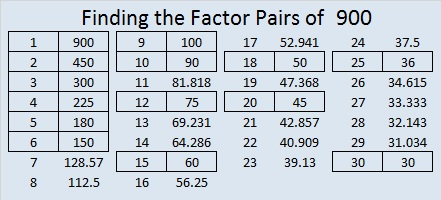# 800 Which Pony Will Take Second Place?

Every 100 posts I summarize the amount of factors of the previous 100 numbers.

MANY of the numbers from 701 to 800 have FOUR factors, and any other number-of-factors doesn’t even come close. For this Horse Race, SECOND place is much more interesting as there are several lead changes. I’ve shorten the track so the second place number-of-factors can reach the finish line.

So go ahead, pick the number-of-factors pony you think will come in SECOND place. Your best bets are 2, 6, 8, 12, 16 OR the second row of 4 factors!

Make your selection, then click on the graphic below to see how your pony does!Now let me tell you a little bit about the number 800.• 800 is a composite number.
• Prime factorization: 800 = 2 x 2 x 2 x 2 x 2 x 5 x 5, which can be written 800 = (2^5) x (5^2)
• The exponents in the prime factorization are 5 and 2. Adding one to each and multiplying we get (5 + 1)(2 + 1) = 6 x 3 = 18. Therefore 800 has exactly 18 factors.
• Factors of 800: 1, 2, 4, 5, 8, 10, 16, 20, 25, 32, 40, 50, 80, 100, 160, 200, 400, 800
• Factor pairs: 800 = 1 x 800, 2 x 400, 4 x 200, 5 x 160, 8 x 100, 10 x 80, 16 x 50, 20 x 40 or 25 x 32
• Taking the factor pair with the largest square number factor, we get √800 = (√400)(√2) = 20√2 ≈ 28.28427.800 is the sum of four consecutive primes:

• 193 + 197 + 199 + 211 = 800

800 is a palindrome in three different bases.

• 2222 BASE 7 because 2(7^3) + 2(49) + 2(7) + 2(1) = 800 (This fact also means that x^3 + x² + x – 399 can be divided evenly by x – 7.)
• 242 BASE 19 because 2(19²) + 4(19) + 2(1) = 800
• PP BASE 31 (P is 25 base 10) because 25(31) + 25(1) = 800

800 is the sum of two squares two different ways:

• 28² + 4² = 800
• 20² + 20² = 800

That being true, it follows that 800 is the hypotenuse of two Pythagorean triples:

• 480-640-800 which is 160 times 3-4-5
• 224-768-800 which is 32 times 7-24-25

800 is also the sum of three squares:

• 20² + 16² + 12² = 800

This chart summarizes the number of factors for the first 800 numbers and indicates that 39% of those numbers have square roots that can be simplified (reduced).In case you didn’t click on the Horse Race image before, here it is, no clicking required: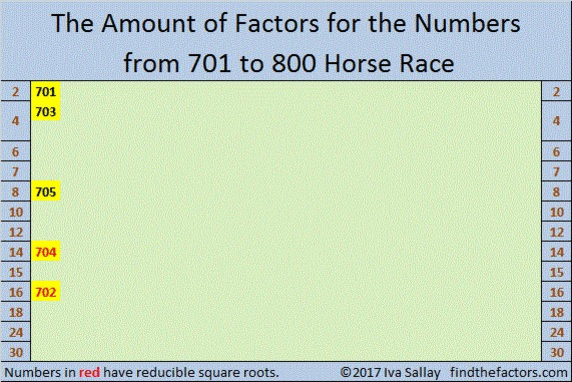make science GIFs like this at MakeaGif

# 700 Pick Your Pony! Who will win this Amount of Factors Horse Race?

• 700 is a composite number.
• Prime factorization: 700 = 2 x 2 x 5 x 5 x 7, which can be written 700 = (2^2) x (5^2) x 7
• The exponents in the prime factorization are 2, 2 and 1. Adding one to each and multiplying we get (2 + 1)(2 + 1)(1 + 1) = 3 x 3 x 2 = 18. Therefore 700 has exactly 18 factors.
• Factors of 700: 1, 2, 4, 5, 7, 10, 14, 20, 25, 28, 35, 50, 70, 100, 140, 175, 350, 700
• Factor pairs: 700 = 1 x 700, 2 x 350, 4 x 175, 5 x 140, 7 x 100, 10 x 70, 14 x 50, 20 x 35 or 25 x 28
• Taking the factor pair with the largest square number factor, we get √700 = (√100)(√7) = 10√7 ≈ 26.457513.Because this is my 700th post, I think I’ll have another horse race. Some numbers from 601 to 700 have exactly 2 factors, 4 factors, and so forth up to 24 factors. (Only perfect squares can have an odd number of factors.)

Which number from 1 to 24 will win this amount of factors horse race? Which number will come in second place, or third place? Cheering for more than one pony will make the race even more interesting.

Here we see that the numbers 2, 6, & 8 are the first ones out of the gate. Click on the graphic to see the rest of this very thrilling horse race:Every hundred posts I also like to focus on the percentage of numbers whose square roots can be simplified.

700 is divisible by 100 so its square root can easily be simplified: √700 = 10√7.

273 of the first 700 numbers have reducible square roots. That’s exactly 39%.

The rest of the numbers, 427, which is 41% of the first 700 numbers, do not have reducible square roots.

Here’s a table breaking down the amount of factors in each group of one hundred integers and the number of reducible square roots.Here are some facts about the number 700.

700 is a palindrome in several bases:

• 4A4 BASE 12; note A is equivalent to 1o in base 10, and 4(144) + 10(12) + 4(1) = 700
• PP BASE 27; note P is equivalent to 25 in base 10, and 25(27) + 25(1) = 700
• KK Base 34; note K is equivalent to 20 in base 10, and 20(34) + 20(1) = 700

700 is the sum of four consecutive prime numbers: 167 + 173 + 179 + 181.

Here is a beautiful painting of a horse race that I saw on twitter: